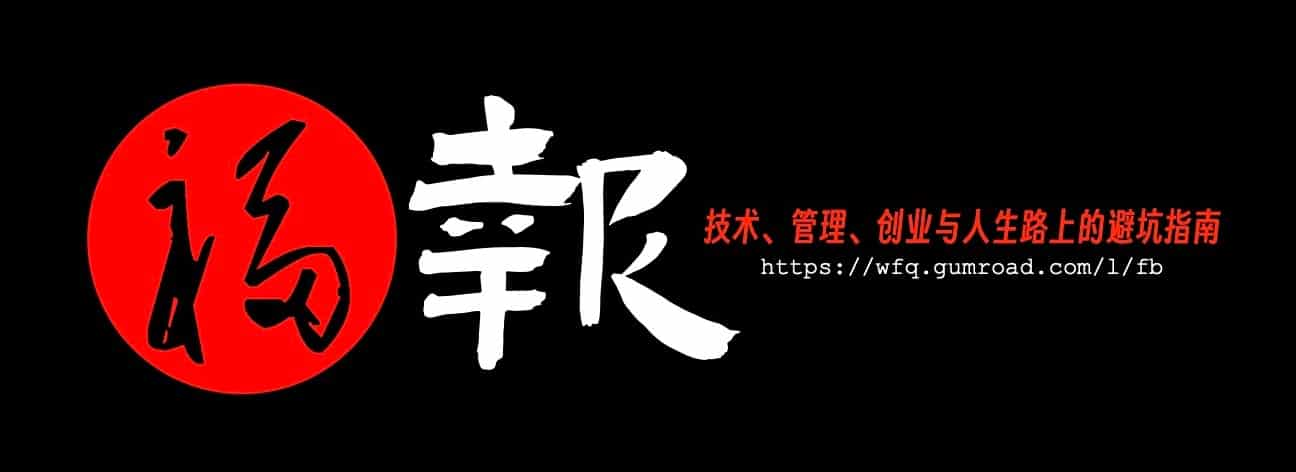# First In, First Out

2014年从msn space存档中重新恢复出来！

```            Suppose that Phil started business on January 1st.  During the year he made the following purchases

January                 260 units @ \$10 per unit = \$2,600

May                       150 units @ \$13 per unit = \$1,950

September             200 units @ \$16 per unit = \$3,200

December              100 units @ \$20 per unit = \$2,000

Total                       710 units                            \$9,750=total purchases for the year

Of these 710 units, suppose 515 were sold during the year leaving 195 units in ending inventory

First in, first out or FIFO:

With FIFO the 515 units sold are assumed to come from the first three purchases made during the year (Jan-Sept).   This leaves all 100 units of the December purchase in inventory and 95 of the September purchase in inventory.   The COGS is calculated as follows:

January                         260 units @ \$10 per unit = \$2,600

Plus May                       150 units @ \$13 per unit = \$1,950

Plus September             105 units @ \$16 per unit = \$1,680

Total                          515 units                              \$6,230= COGS

This means the valuation for the ending inventory is

September                      95 units @ \$16 per unit = \$1,520

Plus December             100 units @ \$20 per unit = \$2,000

Total                          195 units                              \$3,520= Ending inventory

Advantage: It more accurately reflects the replacement cost of inventory items during periods of inflation.

Disadvantage: It reduces the cost of goods sold during inflationary periods thereby increasing company income tax liability.

Last in, first out or LIFO:

With LIFO the 515 units sold are assumed to come from the last three purchases made during the year (Sept-Dec) and an additional 65 units from the January purchase.

The COGS is calculated as follows:

January                           65 units @ \$10 per unit =    \$650

Plus May                       150 units @ \$13 per unit = \$1,950

Plus September              200 units @ \$16 per unit = \$3,200

Plus December              100 units @ \$20 per unit = \$2,000

Total                              710 units                             \$7,800=COGS

This means the valuation for the ending inventory is

January                         195 units @ 10 per unit = \$1,950

Total                          195 units                           \$1,950= Ending inventory

Note:

Cost of goods available for sale (LIFO) = COGS + Ending inventory = 7,800 + 1,950 = 9,750

Cost of goods available for sale (FIFO) = COGS + Ending inventory = 6,230 + 3,520 = 9,750

其中的COGS是Cost Of Goods Sold的缩写。
```

「为AI疯狂」星球上，扶墙老师正在和朋友们讨论有趣的AI话题，你要不要⼀起来呀？^-^

1. 不但有及时新鲜的AI资讯和深度探讨
2. 还分享AI工具、产品方法和商业机会
3. 更有体系化精品付费内容等着你，加入星球(https://t.zsxq.com/0dI3ZA0sL) 即可免费领取。(加入之后一定记得看置顶消息呀！)